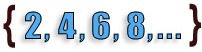# Sets and Set Theory

This instructional unit on sets provide a step-by-step introduction to sets and set theory. Basic definitions and notation, types of sets, equality, and Venn Diagrams are presented. This unit also covers subsets, the Universal set, set-builder notation, complement, intersection and union. A solid foundation on sets is provided for students of all ages. Connections to other disciplines and to the real world are made throughout.Lessons on Sets Description Introduction Students learn that a set is a collection of objects (elements) that have something in common. We define a set by listing or describing its elements. Basic Set Notation Basic notation is used to indicate whether or not an element belongs to a set. Connections are made to language arts, science and social studies. Types of Sets Students learn about finite and infinite sets, as well as the empty or null set. Roster notation is used. Connections are made to art, science, and language arts. Set Equality Students learn how to determine if two sets are equal. The order in which the elements appear in the set is not important. Real-world connections are made with sets. Venn Diagrams Venn diagrams are used to represent sets pictorially, and to show relationships and logical relationships between sets. Intersection and union of overlapping sets are introduced. Subsets Venn diagrams are used to show subsets, with one set contained within the other. The distinction between subsets and proper subsets is made. The relationship between equal sets and subsets is presented, as well as how to determine the number of subsets a given set can have. Universal Set The Universal set is presented as the set of all elements under consideration. Complete Venn diagrams are used to represent sets which are disjoint, overlapping, or one contained within another. Real-world connections are made. Set-Builder Notation Set-builder notation is introduced as a shorthand for writing sets, including formulas, notation and restrictions. Common types of numbers are defined, including natural numbers, integers, and real and imaginary numbers. Students are shown why they need set-builder notation. Complement The complement of a set is defined and shown through numerous examples. Alternate  notations for complement are presented. Set-builder notation and Venn diagrams are included. Connections are made to the real world. Intersection The intersection of two sets is defined and shown through examples with Venn diagrams. Examples include overlapping sets, disjoint sets, and subsets. Procedures for drawing intersections are provided. Real-world connections are made. Union The union of two sets is defined and shown through examples with Venn diagrams. Examples include overlapping sets and subsets. Intersection and union of sets are compared and contrasted. Connections are made to the real world. Practice Exercises Students complete 10 additional exercises as practice, and assess their understanding of all concepts learned in this unit. Challenge Exercises Students solve 10 problems that challenge their understanding of sets and set theory. They hone their problem-solving skills as well. Solutions Complete solutions are provided for all exercises presented in this unit. The problem, step-by-step solutions, and final answer for each exercise are provided. Learning Objectives Set Theory Definitions And Notation, Types Of Sets, Equality, Venn Diagrams, Subsets, Universal Set, Set-Builder Notation, Complement, Intersection And Union..